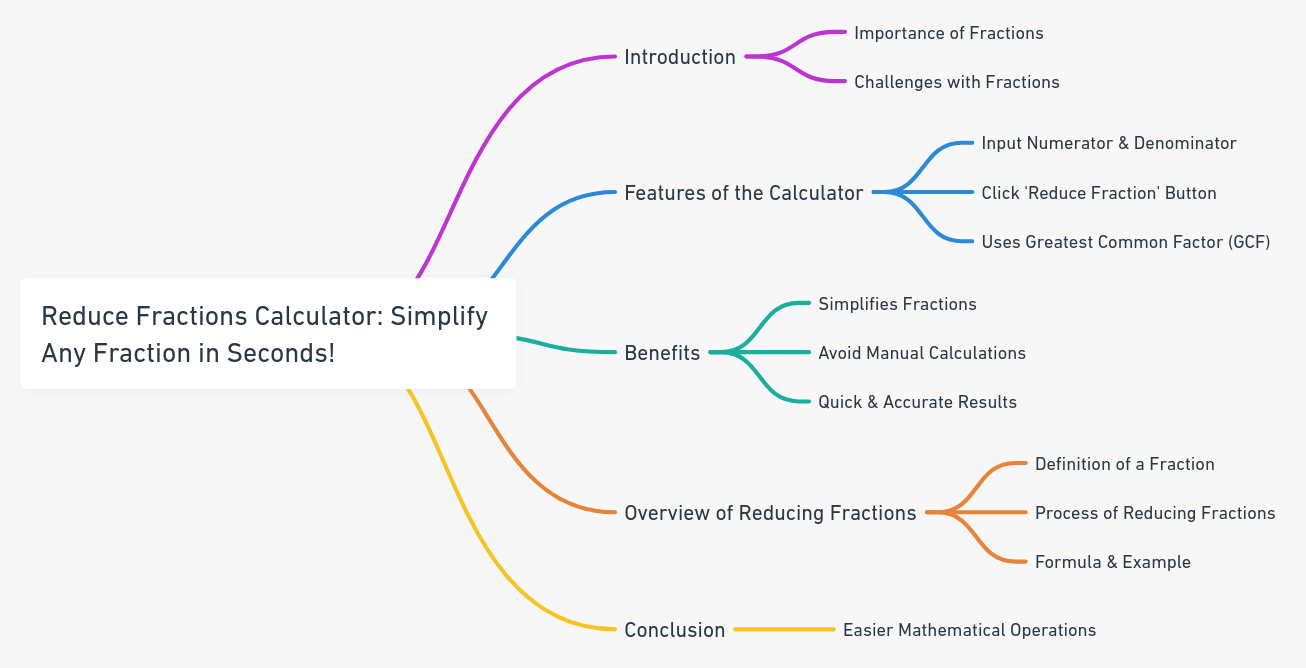Even The mini Tools Can Empower People to Do Great Things.

# Reduce Fractions Calculator: Simplify Any Fraction in Seconds!

Discover our enhanced Reduce Fractions Calculator. Easily simplify any fraction to its lowest terms with step-by-step guidance.

Reduce Fractions Calculator
 Numerator: Denominator:

Embed Reduce Fractions Calculator WidgetFractions, though a fundamental concept in mathematics, can sometimes be a bit tricky. Whether you're a student grappling with homework, a teacher preparing lesson plans, or just someone looking to simplify a fraction for daily use, the Reduce Fractions Calculator is here to help!

The Reduce Fractions Calculator is an invaluable tool designed to simplify any fraction to its lowest terms. By entering the numerator and denominator into the designated boxes and clicking the "Reduce Fraction" button, the calculator effortlessly divides both numbers by their Greatest Common Factor (GCF), presenting the fraction in its most straightforward form. No more manual calculations or guesswork – achieve accurate results in mere seconds!

## Reduce Fractions: An Overview

Fractions represent a part of a whole. In mathematics, a fraction is expressed as a ratio of two numbers: the numerator (the top number) and the denominator (the bottom number).

Reducing fractions, also known as simplifying fractions, is the process of expressing a given fraction in its simplest form. This means that the numerator and the denominator have no common factors other than 1.

### Formula for Reducing Fractions:

To reduce a fraction to its simplest form:

1. Find the Greatest Common Factor (GCF) of the numerator and the denominator.
2. Divide both the numerator and the denominator by the GCF.

Example:

1. Consider the fraction 8/12.
2. The GCF of 8 and 12 is 4.
3. Divide both the numerator (8) and the denominator (12) by 4.
4. The reduced fraction is 2/3.

By reducing fractions to their simplest form, mathematical operations like addition, subtraction, multiplication, and division become more straightforward, and the results are easier to interpret.